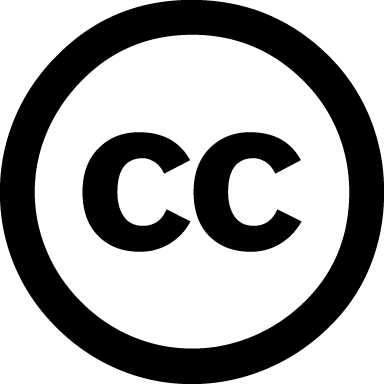https://www.dagstuhl.de/13411

# Deduction and Arithmetic

## Organizers

Nikolaj S. Bjørner (Microsoft Corporation – Redmond, US)
Reiner Hähnle (TU Darmstadt, DE)
Tobias Nipkow (TU München, DE)
Christoph Weidenbach (MPI für Informatik – Saarbrücken, DE)

## Documents

Dagstuhl Report, Volume 3, Issue 10Aims & Scope
List of Participants
Dagstuhl's Impact: Documents available
Dagstuhl Seminar Schedule [pdf]

## Summary

Arithmetic plays a fundamental role in deduction. Logical constraints over arithmetical properties occur frequently in classical theorems in mathematics, as well as in program analysis and verification. The first automatic theorem prover was an implementation of Presburger Arithmetic in 1954. With the availability of powerful predicate calculus proof procedures some years later, arithmetic would be relegated to the sidelines. Interest in arithmetic revived in the 1980s with the advent of powerful interactive theorem provers that needed and supported arithmetic for their applications. The need for efficient computer aided deduction with support for arithmetic in the area of program analysis and verification recently gave birth to a new technology, so called SMT solvers.

Thus we have three strands of automated deduction: SMT solvers, automated first-order provers, and interactive provers in need of (more) arithmetic.

SMT SMT (satisfiability modulo theories) solvers distinguish themselves by integrating built-in support for a combination of theories, including prominently the theory of arithmetic. Most often handling arithmetic formulas in isolation is not sufficient. Applications typically use a non-disjoint combination of arithmetic and other theory reasoning. SMT solvers nowadays handle quantifier-free arithmetic well, but are not directly equipped to solve arithmetical formulas with quantifiers. Recent progress on building in quantifier-elimination procedures for linear and non-linear arithmetic have made practical integration of such richer arithmetic deduction viable.

ATP Research in first-order logic theorem proving used to concentrate on efficient calculi in general and the integration of equational theories in particular. It is obvious that further integration of ``richer'' arithmetic theories into first-order logic should be done by rather a combination approach than an integration approach. One major challenge of combining first-order logic calculi with arithmetic procedures is that of compactness/completeness and termination. While Boolean combinations of ground atoms, as they are considered by SMT solvers typically do not cause trouble with respect to those challenges, combining first-order clauses with an arithmetic theory can never result in a compact/complete/terminating calculus, in general. The actual combination typically requires the solution of purely arithmetic problems in order to establish valid inferences and simplifications. These problems are of a specific nature in that the form of the arithmetic formulas and the way they need to be tested require specific variants of the known arithmetic procedures.

ITP Interactive theorem provers initially came with built-in decision procedures for quantifier-free linear arithmetic. More foundational systems then developed new techniques to implement these decision procedures by reducing them to pure logic, trading efficiency for guaranteed correctness. Aspects of arithmetic reasoning are present in deductive software verification systems: interactive systems combine a number of automatic arithmetic reasoning methods and control them with heuristics that are specific for verification. A challenging application of interactive proof and arithmetic is the Flyspeck project, an effort to formalize Hales's proof of the Kepler conjecture in an interactive theorem prover.

The Dagstuhl seminar was a timely event that brought together experts in the above subareas of deduction, and in reasoning about arithmetic, to exchange experiences and insights. The research questions pursued and answered included:

• Which arithmetic problems are best solved with which approach?
• How to handle very complex numeric representations such as the IEEE floating-point standard with a high degree of automation?
• Arithmetic in combination with other theories results easily in languages with a very complex decision problem---how can a high degree of automation be obtained nevertheless?
• How can SMT-based reasoning be combined with model-based reasoning?
• What is the best way to incorporate arithmetic simplification available in computer algebra systems into deductive frameworks?
• How can the specific structure of arithmetic problems generated by deduction systems be exploited?

In addition to the technical contributions, the seminar participants attempted in an open discussion session to identify the major trends and open questions around Deduction and Arithmetic.Creative Commons BY 3.0 Unported licenseNikolaj S. Bjørner, Reiner Hähnle, Tobias Nipkow, and Christoph Weidenbach

## Classification

• Artificial Intelligence
• Semantics / Formal Methods
• Verification / Logic

## Keywords

• Automated Deduction
• Program Verification
• Arithmetic Constraint Solving

## Documentation

In the series Dagstuhl Reports each Dagstuhl Seminar and Dagstuhl Perspectives Workshop is documented. The seminar organizers, in cooperation with the collector, prepare a report that includes contributions from the participants' talks together with a summary of the seminar.

## Dagstuhl's Impact

Please inform us when a publication was published as a result from your seminar. These publications are listed in the category Dagstuhl's Impact and are presented on a special shelf on the ground floor of the library.

## Publications

Furthermore, a comprehensive peer-reviewed collection of research papers can be published in the series Dagstuhl Follow-Ups.